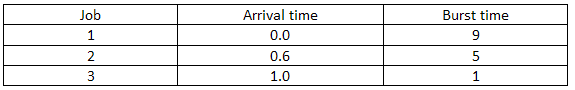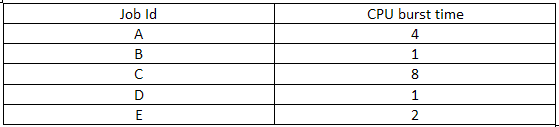# Placement papers on Operating System - Set 13

## Placement papers on Operating System - Set 13

Q1. Priority is assigned by the scheduling algorithm in proportional to the waiting time of a process. Each and every process starts with the lowest priority i.e zero priority. After

every T time units the schedule reevaluates the priority of process and decided the next process to be scheduled. This is equivalent to which algorithm if the process have no I/O operations

and all arrive at the time zero?

1. Shortest algorithm remaining time first
2. Shortest job first
3. Round-Robin algorithm
4. First come first serve

Q2. _______________does not interrupt a running process

1. Scheduler process
2. A device
3. Power failure
4. Timer

Q3. Example of spooled device is

1. The swapping area on a disk used by the swapper.
2. The secondary memory device in a virtual storage system.
3. An output device used to print the output of a number of jobs.
4. The terminal used to enter the input data for the c program being executed.

ANSWER: 3. An output device used to print the output of a number of jobs.

Q4. Which sequence is an optimal non-preemptive scheduling sequence for the following jobs which leaves the CPU idle for 1 unit of time?1. {2, 1, 3}
2. {1, 2, 3}
3. {3, 2,1}
4. None of the above

Q5. The jobs given in the table below are executed on a single processor system.Assuming that the order of jobs arrival is A, B, C, D, E at time 0?. If Round robin scheduling algorithm with time slice of 1 is used then what is the departure time or completion

time for job A?

1. 15
2. 12
3. 10
4. 11